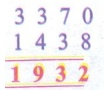Home | | Maths 4th Std | Exercise 2.3c (Subtraction with Regrouping)

# Exercise 2.3c (Subtraction with Regrouping)

Text Book Back Exercises Questions with Answers, Solution : 4th Maths : Term 1 Unit 2 : Numbers : Exercise 2.3c (Subtraction with Regrouping)

Exercise 2.3c

A. SubtractB. Find the difference between given numbers.

a) 4352 and 5020

b) 1438 and 3370

c) 2526 and 8431

d) 3361 and 9000

a) 4352 and 5020Difference = 668

b) 1438 and 3370Difference = 1932

c) 2526 and 8431Difference = 5905

d) 3361 and 9000Difference = 5639

1. The sum of two numbers is 7036, one number is 3168. Find the other number.

Sum of two numbers = 7036 (−)

Given number           = 3168 (Subtract this from 7036)

The other number is 3868

2. A man had Rs 9200 in the bank. He withdrew Rs 2756. How much money does he have in the bank now?

Amount in the bank = 9200 (−)

Amout withdrawn = 2756

Amount in the bank now = 6444

He have Rs. 6444 in the bank now.

D. Create the subtraction story problems for the details given below.

a) 1997 – 1968(a) A volcano in Japan erupted in 1968 and in 1997. After how many years did it erupt?

(b) The yield of mangoes in a field was 1818 kg in 2010. In 2019 it was 1011kg. How much was the yield lower in 2019 than in 2010?

(c) The export of banana was 2018 tonnes last year. This year it was only 915 tonnes. How much is the export lower this year?

Activity

Number puzzle

Solve the subtraction problems. Now write the differences. One has been done for you.Tags : Numbers | Term 1 Chapter 2 | 4th Maths , 4th Maths : Term 1 Unit 2 : Numbers
Study Material, Lecturing Notes, Assignment, Reference, Wiki description explanation, brief detail
4th Maths : Term 1 Unit 2 : Numbers : Exercise 2.3c (Subtraction with Regrouping) | Numbers | Term 1 Chapter 2 | 4th Maths